7:48 PM
Transforming Energy into Mass: Particle Creation Michael Fowler, University of Virginia

# Transforming Energy into Mass: Particle Creation

Michael Fowler, University of Virginia

### Pion Production

We have mentioned how, using a synchrocyclotron, it is possible to accelerate protons to relativistic speeds.  The rest energy of a proton mpc2 is 938 MeV, using here the standard high energy physics energy unit: 1 MeV = 106 eV.  The neutron is a bit heavier—mnc2 = 940 MeV.  (The electron is 0.51 MeV).  Thus to accelerate a proton to relativistic speeds implies giving it a K.E. of order 1,000 MeV, or 1 GeV.

The standard operating procedure of high energy physicists is to accelerate particles to relativistic speeds, then smash them into other particles to see what happens.  For example, fast protons will be aimed at protons at rest (hydrogen atoms, in other words—the electron can be neglected).  These proton-proton collisions take place inside some kind of detection apparatus, so the results can be observed.  One widely-used detector is the bubble chamber: a transparent container filled with a superheated liquid.  The electric field of a rapidly moving charged particle passing close to a molecule can dislodge an electron, so an energetic particle moving through the liquid leaves a trail of ionized molecules. These give centers about which bubbles can nucleate.  The bubbles grow rapidly and provide a visible record of the particle’s path.

What is actually observed in p-p scattering at relativistic energies is that often more particles come out than went in—particles called pions, p+, p0, p- can be created.  The p0 is electrically neutral, the p+ has exactly the same amount of charge as the proton.  It is found experimentally that total electric charge is always conserved in collisions, no matter how many new particles are spawned, and total baryon number (protons + neutrons) is conserved.

Possible scenarios include: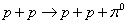and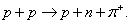The neutral pion mass is 135 MeV, the charged pions have mass 140 MeV, where we follow standard high energy practice in calling mc2 the “mass”, since this is the energy equivalent, and hence the energy which, on creation of the particle in a collision, is taken from kinetic energy and stored in mass.

However, an incoming proton with 135 MeV of kinetic energy will not be able to create a neutral pion in a collision with a stationary proton.  This is because the incoming proton also has momentum, and the collision conserves momentum, so some of the particles after the collision must have momentum and hence kinetic energy.  The simplest way to figure out just how much energy the incoming proton needs to create a neutral pion is to go to the center of mass frame, where initially two protons are moving towards each other with equal and opposite velocities, there is no total momentum.  Obviously, in this frame the least possible K.E. must be just enough to create the p0 with all the final state particles (p,p,p0) at rest. Thus if the relativistic mass of the incoming protons in the center of mass frame is m, the total energy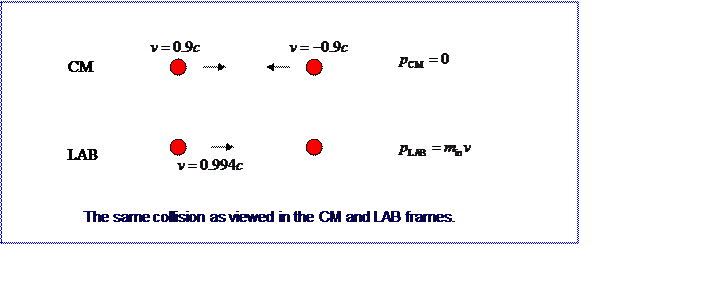Putting in the proton and pion masses from above, and using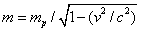, we find the two incoming protons must both be traveling at 0.36c

Recall that this is the speed in the center of mass frame, and for practical purposes, like designing the accelerator, we need to know the energy necessary in the “lab” frame—that in which one of the protons is initially at rest.  The two frames obviously have a relative speed of 0.36c, so to get the speed of the incoming proton in the lab frame we must add a velocity of 0.36c to one of 0.36c using the relativistic addition of velocities formula, which gives 0.64c.  This implies the incoming proton has a relativistic mass of 1.3 times its rest mass, and thus a K.E. around 280 MeV.

Thus to create a pion of rest energy 135 MeV, it is necessary to give the incoming proton at least 290 MeV of kinetic energy.  This is called the “threshold energy” for pion production.  The “inefficiency” arises because momentum is also conserved, so there is still considerable K.E. in the final particles.

### Antiproton Production

On raising the energy of the incoming proton further, more particles are produced, including the “antiproton”—a negatively charged heavy particle which will annihilate a proton in a flash of energy.  It turns out experimentally that an antiproton can only be produced accompanied by a newly created proton,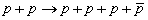Notice we could have conserved electric charge with less energy with the reaction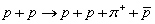but this doesn’t happen—so charge conservation isn’t the only constraint on which particles can be produced.  In fact, what we are seeing here is experimental confirmation that the conservation of baryon number, which at the low energies previously discussed in the context of pion production just meant that the total number of protons plus neutrons stayed fixed, is generalized at high energies to include antiparticles with negative baryon number, -1 for the antiproton. Thus baryon number conservation becomes parallel to electric charge conservation, new particles can always be produced at high enough energies provided the total new charge and the total new baryon number are both zero. (Actually there are further conservation laws which become important when more exotic particles are produced, which we may discuss later.)  We should emphasize again that these are experimental results gathered from examining millions of collisions between relativistic particles.

One of the first modern accelerators, built at Berkeley in the fifties, was designed specifically to produce the antiproton, so it was very important to calculate the antiproton production threshold correctly!  This can be done by the same method we used above for pion production, but we use a different trick here which is often useful.  We have shown that on transforming the energy and momentum of a particle from one frame to another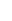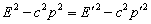Since the Lorentz equations are linear, if we have a system of particles with total energy E and total momentum p in one frame, E' , p' in another, it must again be true that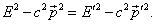We can use this invariance to get lab frame information from the center of mass frame.  Noting that in the center of mass (CM) frame the momentum is zero, and in the lab frame the momentum is all in the incoming proton,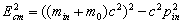where here m0 is the proton rest mass, min is the relativistic mass of the incoming proton.  At the antiproton production threshold, Ecm = 4m0c2, so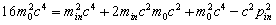and using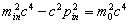we find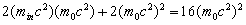,

so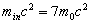.

Therefore to create two extra particles, with total rest energy 2m0c2, it is necessary for the incoming proton to have a kinetic energy of 6m0c2.  The Berkeley Gevatron had design energy 6.2 GeV.

As we go to higher energies, this “inefficiency” gets worse—consider energies such that the kinetic energy >> rest energy, and assume the incoming particle and the target particle have the same rest mass, m0, with the incoming particle having relativistic mass m.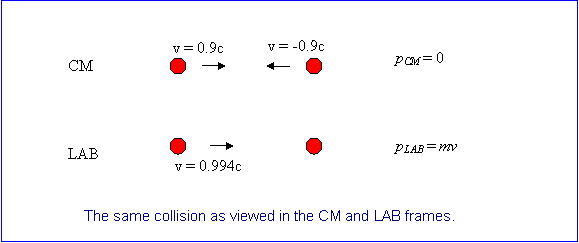Comparing the center of mass energy with the lab energy at these high energies,For m >> m0,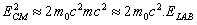so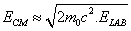,

ultimately one must quadruple the lab energy to double the center of mass energy.

Category: Education | Views: 694 | Added by: farrel | | Rating: 0.0/0